# R for Researchers: Data exploration

This article is part of the R for Researchers series. For a list of topics covered by this series, see the Introduction article. If you're new to R we highly recommend reading the articles in order.

## Overview

This article will introduce you to some common functions used to explore a data set numerically and graphically. One of the important tools introduced in this article is the ggplot set of functions used for graphing. The ggplot functions will be used in several other articles in this series.

This article will demonstrate the R functions using the Salary data set created in the Data preparation article. The focus is on the use of R for data exploration, not the statistical methods which might be used. As such only minimal comments will be made on the interpretation of the results.

## Preliminaries

You will get the most from this article if you follow along with the examples in RStudio. Working the exercise will further enhance your skills with the material. The following steps will prepare your RStudio session to run this article's examples.

• Start RStudio and open your RFR project.
• Confirm that RFR (the name of your project) is displayed in the upper left corner of the RStudio window.
• Open your SalAnalysis script.
• Run all the commands in SalAnalysis script.

## Numeric representations

We will start by adding a code block to demarcate the start of the exploration section of our script.

• Enter the following commands at the bottom of your script and run them.

#####################################################
#####################################################
##
##   Exploration section
##
#####################################################
#####################################################

### Summary statistics

Summary statistics are a good place to start when looking at a new dataset. The summary() function provides the summary of each variable in a data.frame.

• The syntax and use of the summary() function

summary(object)

Returns a table with a column for each of the variables in a data.frame.

For factor, logical, and character variables, the frequency of occurrences is returned. Low frequency levels will be combined in an "other" category if there are too many levels to be displayed.

For numeric variables, the five number summary (median, first quartile, third quartile, min, and max) and the mean are returned.

We will look at the summary of the salary dataset.

• Enter the following command in your script and run it.

summary(salary)
• The results are shown below.

        rank     dscpl       yrSin           yrSer           sex
AssocProf: 64   A:181   Min.   : 1.00   Min.   : 0.00   Female: 39
AsstProf : 67   B:216   1st Qu.:12.00   1st Qu.: 7.00   Male  :358
Prof     :266           Median :21.00   Median :16.00
Mean   :22.31   Mean   :17.61
3rd Qu.:32.00   3rd Qu.:27.00
Max.   :56.00   Max.   :60.00
salary          logSal         salLev
Min.   : 57.8   Min.   :4.057   high  :100
1st Qu.: 91.0   1st Qu.:4.511   low   : 98
Median :107.3   Median :4.676   middle:199
Mean   :113.7   Mean   :4.700
3rd Qu.:134.2   3rd Qu.:4.899
Max.   :231.5   Max.   :5.445               
• The range of values seems to be reasonable for college professors.

## Correlation between variables

Another useful method to explore the relationships within a dataset is to examine the correlation between the variables.

• The syntax and use of the cor() function

cor(object)

Returns a matrix of the correlations.

The object passed to cor needs to be a two dimensional object with a type of numeric.

The salary data.frame is a two dimensional object, though not all the variables are numeric. We will need to change the type of the non-numeric variables to numeric or exclude the non-numeric variables from our call to cor().

What we have found is that we need to do some data preparation work as we are exploring the data. This is a fairly normal occurrence. It is best to keep the code that does data preparation together. The following code to create a numeric data.frame should be added to the data preparation section of the script.

We will change the factor variables to type numeric. To convert a factor to a meaningful numeric variable there needs to be a meaningful order to the levels. In our example we will need the levels of professor rank to be ordered according to increasingly higher levels of rank. We will use the levels parameter of the factor function for this.

• The syntax and use of the levels parameter to factor()

factor(variable,levels=levelsVector)

The order of the levels in levelsVector is the order of the level values used in the factor variable.

The first level in levelsVector will be the reference level.

We will order the ranks from lowest rank to highest rank.

• Enter the following command in your script and run it.

salary$rank <- factor(salary$rank,
levels = c("AsstProf","AssocProf","Prof")
)
• There are no console results from this command.

We do not want to permanently change the variables in salary. So we will make a copy of the salary data.frame, called salaryNum, to make the variables numeric.

• Enter the following command in your script and run it.

salaryNum <- salary

The change of type can be done to each variable individually. For a data set of this size that would be fairly easy to do, but we will do it with a for loop.

• The syntax and use of for loop

for (*var* in *vector*) {
*repeatedCommands*
}  

The set of repeatedCommands will be run once for each element in vector.

var will be set to successive values of the elements of vector on each iteration of the loop.

• Enter the following commands in your script and run them.

for (i in colnames(salary)) {
salaryNum[,i] <- as.numeric(salary[,i])
}

Now that we have the dataset in the form we need, we can use the cor() function.

• Enter the following command in your script and run it.

round( cor(salaryNum[,-c(8)]), 3)
• The results of the above command is shown below

         rank  dscpl  yrSin  yrSer   sex salary logSal
rank    1.000 -0.107  0.701  0.608 0.139  0.617  0.667
dscpl  -0.107  1.000 -0.218 -0.165 0.004  0.156  0.174
yrSin   0.701 -0.218  1.000  0.910 0.149  0.419  0.426
yrSer   0.608 -0.165  0.910  1.000 0.154  0.335  0.341
sex     0.139  0.004  0.149  0.154 1.000  0.139  0.148
salary  0.617  0.156  0.419  0.335 0.139  1.000  0.988
logSal  0.667  0.174  0.426  0.341 0.148  0.988  1.000

From the correlation table, the column for log salary values are a little higher than those of salary. This indicates that the data may be a little better fit to log salary than to salary. The correlation between yrs.since.phd and yrs.service is about .9. This correlation is not surprising.

### Group sizes

Group size is an important consideration in regression. We will look at the group sizes using two different functions. The first is table(), which makes a frequency table.

• The syntax and use of the table() function

table(vector1,vector2,...,vectorn)

Returns a table of the frequency counts of combinations of groups from the vectors. If 2 vectors are provided, a two by two contingency table is created.

Each of the vectors needs to be a grouping variable. A grouping variable is a variable of type factor or a variable whose values identify groups of interest.

Lets look at the group sizes formed by unique combinations of rank, discipline, and sex.

• Enter the following command in your script and run it.

table(salary$rank,salary$dscpl,salary$sex)  • The results are shown below. , , = Female A B AsstProf 6 5 AssocProf 4 6 Prof 8 10 , , = Male A B AsstProf 18 38 AssocProf 22 32 Prof 123 125 From the results you can see that the table R constructed is 3 dimensional. There is one dimension for each of the grouping variables used in the table() function. Table() is convenient if there are one or two grouping variables of interest. We also will use the aggregate function to get the size of the groups. • The syntax and use of the aggregate() function aggregate(aggVec, by = list(grpVec1,...,grpVecn), FUN = function ) Returns a data.frame. There is a row for each unique combinations of groups in the list of grpVec. There is a column for each grpVec in the list. There is also a column with the frequency count for each of the unique combinations. AggVec is the variable with the elements which will be aggregated using function. A multi-column structure can also be used for AggVec and each column of the structure will be aggregated. The by list is used to determine what subsets of the aggVec the function is applied to. Each grpVec in the list is a grouping variable. Function is any R function which takes a vector as its parameter and returns a single value. This is typically a summary statistic function such as mean, sum, etc. To use aggregate, we need a function which will give us the number of elements in a group. We will use the length() function. • The syntax and use of the length() function length(vector) Returns the number of elements of vector Putting the aggregate() and length() functions together we can get the desired frequency counts. • Enter the following command in your script and run it. aggregate(salary$salary,
by = list(rank=salary$rank, dscpl=salary$dscpl,
gender=salary\$sex
),
FUN = length
)
• The results of the above command are shown below.

        rank dscpl gender   x
1   AsstProf     A Female   6
2  AssocProf     A Female   4
3       Prof     A Female   8
4   AsstProf     B Female   5
5  AssocProf     B Female   6
6       Prof     B Female  10
7   AsstProf     A   Male  18
8  AssocProf     A   Male  22
9       Prof     A   Male 123
10  AsstProf     B   Male  38
11 AssocProf     B   Male  32
12      Prof     B   Male 125

As you can see from the output, both functions return the same frequency counts for each group. The table() function is the quickest way to get a look at frequency counts of groups. The table() function also produces a good visual representation of the frequency counts. The aggregate() function has greater flexibility in what it can do. The single column form of the aggregate() results is useful if further programming is to be done with the frequency counts.

#### Exercise

These exercises use the alfalfa dataset and the work you started on the alfAnalysis script. Open the script and run all the commands in the script to prepare your session for these problems.

1. Do a summary of the data.frame alfalfa.

2. Do a cor of the shade, irrig, and yield variables. Use only the variables which have a sensible order.

3. Create a frequency table for shade and irrig.

4. Use aggregate to find the mean of the inoculum groups.

5. Commit your changes to AlfAnalysis.

Solutions

## Graphical representations

There are several graphics packages available in R. We will primarily be using the ggplot graphics functions from the ggplot2 package. We will also use a few object-specific plotting functions. These object-specific plots are quick and useful. They are designed to give you a quick look at relationships which are of common interest. These plots will be introduced as they are used in the articles. The object-specific plot functions are typically built on the base graphics functions, the plotting packages included in the core R packages. No background on the base graphics package is needed to use these object specific plotting functions, they typically only need to be given the object to make the desired plot.

The ggplot package is a more current graphics package than the base graphics package. It is based on the grammar of graphics which provides some structure to how plots are specified. The structure of ggplot will be introduced first and then examples will be provided. The examples will show how easy it is to use ggplot(). So push ahead even if the definitions seem a little difficult.

There are minimally four objects which are needed to produce a plot with ggplot.

1. An empty plot. This is the canvas on which the plot will be created. This is an object in R. Like all R objects it can be be given a name through the assignment operator. This allows multiple plots to be worked on in parallel and plots to be refined later.
2. A data.frame containing the data to be plotted.
3. The geometric object to be plotted. This may be points, lines, bars, etc.
4. Aesthetics which provide the information needed to plot the geometric objects, such as x and y locations for points or lines.

To start a plot you create the empty plot. This is the canvas on which the plot will be constructed. The ggplot() function is used to create the empty plot.

• The syntax and use of the ggplot() function

ggplot(data = data.frame, aes(parameters))

Returns a list of plot objects. If the ggplot() function is on the right side of an assignment statement, the list is saved as an object and no plot is generated. If the ggplot() object is passed to the print function (typically through an implied print) the plot is generated.

The returned object does not have a geometry and will not produce a plot on its own.

The data.frame identifies the source of the data which will be used in the plot. The data.frame parameter can be any object which can be coerced to a data.frame.

The aesthetics in the aes parameters are described below. Aesthetics which are declared in the ggplot() function apply to all graphed objects, the scope of the aesthetic is global.

Aesthetics are parameters which control where an object is graphed and the how the graphed object will look. Some of the common aesthetics parameters are

• x is the x position
• y is the y position
• color is the outline color
• fill is the inside color
• shape is the shape of what is to be plotted
• size is the size of the plotted shape object
• linetype is the line type (solid, dashed, etc.) for line objects

A single value can be used for each of these aesthetics and this value will be applied to all the objects within its scope. Setting color, fill, shape, or size to a grouping variable will result in a unique aesthetics for each group.

• An example of the aes() parameter.

aes(x = myX, y = myY, color = "red")

This would add objects with the x and y positions determined by the variables myX and myY and the objects would all have an outline color of red.

Graphical objects are added in layers. Each of these layers will add geometric objects on top of what has already been drawn. A layer may hide (plot over top of) objects plotted in prior layers. Lower layer objects being hidden is typically only an issue in complicated graphs, which are not typically needed. Some of the common geometric objects are:

• geom_point()
• geom_line()
• geom_histogram()
• geom_bar()
• geom_boxplot()

Each of these is a function which will add the geometric shape implied by the function's name.

• The syntax and use of the geom_object() functions

geom_object(aes(parameters))

Where geom_object is one of the geom's in the above list.

Used with the ggplot() function. This function is added to an expression which starts with ggplot().

The function modifies the layer element of the ggplot() object list. This modification of the layer element is its typical returned value.

Geom aesthetics are specified in the geom_object() function in the same way as they are in ggplot(). Geom aesthetics will have a local scope. That is they will only apply to this geom. Local aesthetics take precedence over globally defined aesthetics.

• An example of the ggplot functions used to create a scatter plot is

ggplot(data=myData, aes(x = myX, y = myY, color = "red")) +
$$\ \ \ \$$geom_point()

Notice, it is the plus sign that is used to add the graph parts together to make the plot.

Theme objects include titles, axes, background colors, grid lines, margins, legends, etc. This is basically everything that is not a graphic object. These can be thought of as objects associated with the canvas or the frame around the canvas. The theme functions we will use to add theme objects are

• ggtitle()
• xlab()
• ylab()
• theme_bw()
• theme()

The formatting options we will use for themes are

• axis.title.x
• axis.title.x
• plot.title
• strip.background
• legend.position

The default theme for ggplot is a dark background with light colored objects. This is not always what is desired. We will be using a white background for the plots we create.

• A white background is produced by adding the theme_bw() function.

theme_bw()

Using ggplot is easier than these definitions make it seem. It will be easy to see what each of these parts do by looking at a few examples. The first example is a scatter plot of salary and years of service.

• Enter the following command in your script and run it.

ggplot(data = salary, aes(x = yrSer, y = salary)) +
geom_point() +
theme_bw() +
ggtitle("Professor's salaries from 2008-9") 

The ggplot() function opens a new canvas, connects the salary data.frame to the canvas. It also sets which variables to use for the x and y location on the graph.

The geom_point() function plots circles, the default shape, at the (x,y) pair locations.

Theme_bw() changes the background color.

The ggtitle() function adds a title to the plot.

• Select the Plots tab in RStudio

• The following graph will be displayed in the Plots tab of RStudio.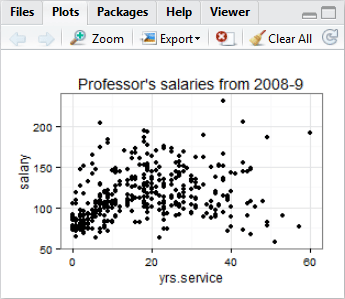Plot tab

The left and right arrows allow you to scroll back and forth through plots you have generated.

The Zoom icon opens the current plot in a separate window. The plot can be rescaled and saved using the plot zoom window.

The export icon opens another window with menu items to assist you in saving the plot.

The plot axis labels could use some improvement and more space between the title and the plot would be nice. We will add functions to provide our own axis labels and provide extra space for the title and axis labels.

• Enter the following commands in your script and run them.

ggplot(data = salary, aes(x = yrSer, y = salary)) +
geom_point() +
theme_bw() +
ggtitle("Professor's salaries from 2008-9") +
theme( plot.title = element_text(vjust=1.0) ) +
xlab("Years of service") +
theme( axis.title.x = element_text(vjust=-.5) ) +
ylab("Salary in thousands of dollars") +
theme( axis.title.y = element_text(vjust=1.0) ) 

The vjust parameter used in the theme functions moves the text objects vertically relative to its orientation. Here positive values moved the the title and y label up, which is away from the graph. The x label had a negative value and moved it down, which is also away from the graph.

• The following plot will be displayed in the Plots tab.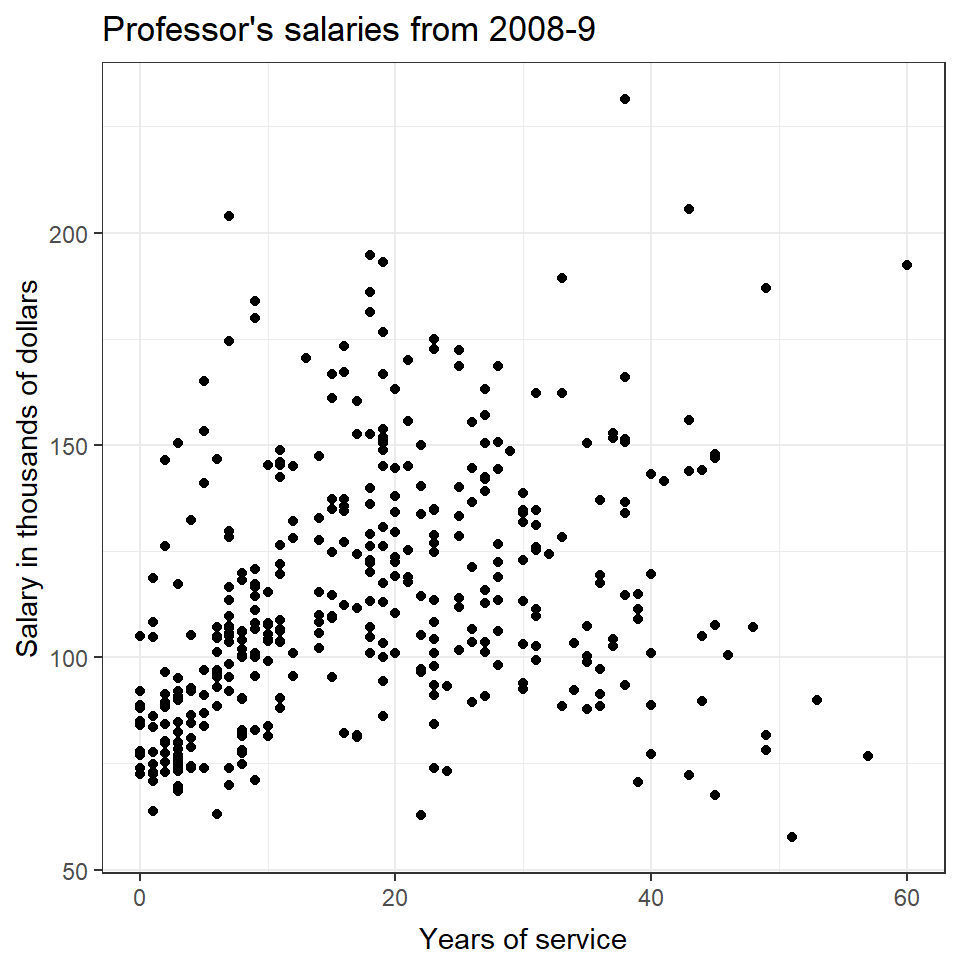This plot required a number of functions to produce it. Each of these functions has a clearly identifiable effect on the plot. So even though there are a number of functions, the code is simple if you remember the basics we covered above.

I am interested in seeing if there is a different relationship between salary and years of service based on rank. I would like to see a separate graph for each rank. This is done in ggplot with facets. Faceting a graph produces a separate plot for each value in a grouped variable. There are two facet functions

• The syntax and use of the facet_grid() and facet_wrap() functions

facet_grid(verticalGroup~horizontalGroup)
facet_wrap(~horizontalGroup)

The facet_grid() function organizes the facets in rows and columns.

The facet_wrap() function organizes the facets in a horizontal row. If there is not enough space to show all the facets on one row, the facets will wrap to the following rows as needed. Hence its name.

We have only one variable, rank, we want to facet on, so we will use facet_wrap. The prior plotting code is used again with the facet added to the plot.

• Enter the following commands in your script and run them.

plotSalFacRank <- ggplot(data = salary, aes(x = yrSer, y = salary)) +
geom_point() +
theme_bw() +
ggtitle("Professor's salaries from 2008-9") +
theme( plot.title = element_text(vjust = 1.0) ) +
xlab("Years of service") +
theme( axis.title.x = element_text(vjust = -.5) ) +
ylab("Salary in thousands of dollars") +
theme( axis.title.y = element_text(vjust = 1.0) ) +
facet_wrap(~rank) +
theme(strip.background = element_rect(fill = "White"))
plotSalFacRank

Notice in the above code the plot object was saved as a variable. This allows the plot object to be used later. To get the plot to be displayed, the implied print command was used.

The theme strip.background was also set to white, the default background for ggplot is dark.

• The following plot will be displayed.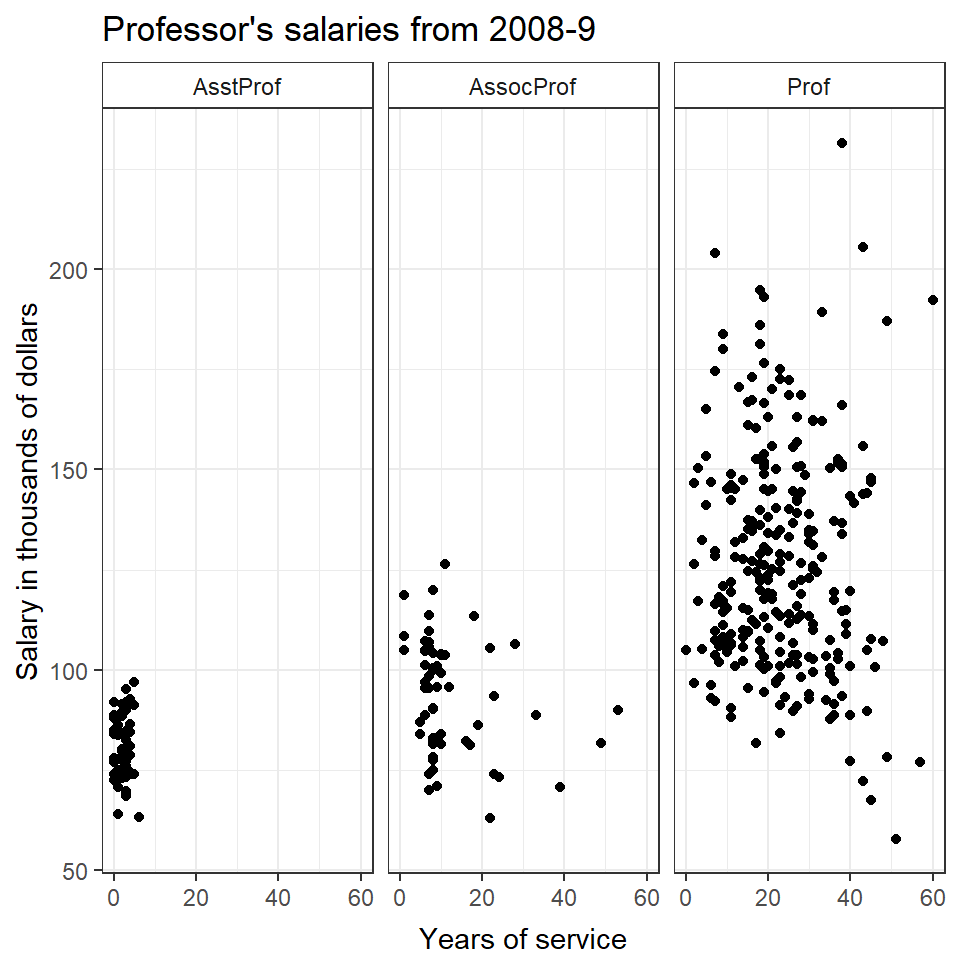Another way this relationship could be visualized is using a different color for each of the ranks. This approach plots all the observations on the same graph. We create this plot by setting the color aesthetic to rank in the geom_point layer. A legend will be created by ggplot for the levels of rank. We will use legend.position parameter to put the legend at the bottom of the plot.

• Enter the following commands in your script and run them.

ggplot(data = salary, aes(x = yrSer, y = salary)) +
geom_point(aes(color = rank)) +
theme_bw() +
ggtitle("Professor's salaries from 2008-9") +
theme( plot.title = element_text(vjust = 1.0) ) +
xlab("Years of service") +
theme( axis.title.x = element_text(vjust = -.5) ) +
ylab("Salary in thousands of dollars") +
theme( axis.title.y = element_text(vjust = 1.0) ) +
theme(legend.position = "bottom")
• The following plot will be displayed.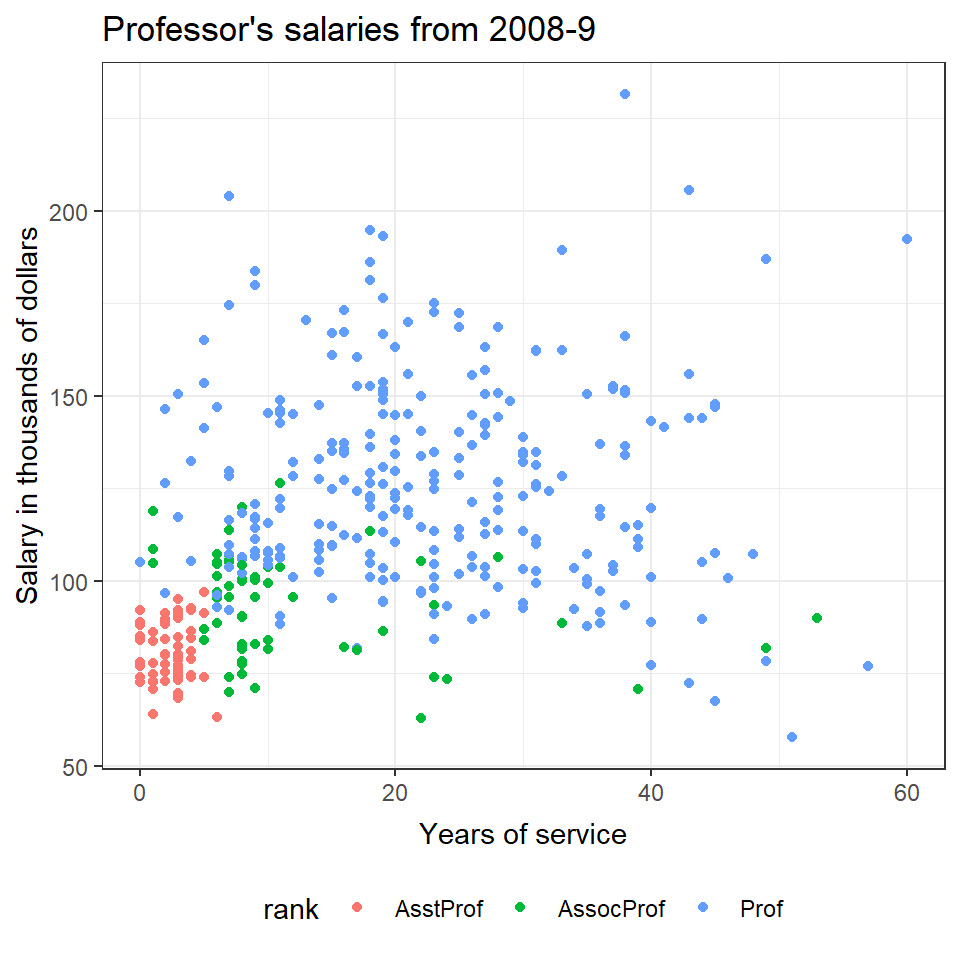The last two graphs were simpler to make using ggplot than they would have been with base graphics. This is typical of using ggplot. That is it will take a few more functions to set up a plot. But, once you have the plot set up in ggplot, it is easy to explore the data in interesting ways. And again, with the background above the functions tie directly to visual effects in the plot.

The final thing you need to know about using ggplot is that it requires your data to be in what is known as long form. A data.frame is in long form if each row is one observation. If you have data in wide form, more than one observation per row, you can change the shape of the data using the reshape() function. Reshape() will be covered in the Regression Diagnostics article.

The plot function from the base graphics package can accept a data.frame as an object to be plotted. It will produce a matrix of pairwise scatter plots of the variables in the data.frame. We will use it to get a quick overview of what relationships we might see in a dataset. Let's use it to take a look at the salary data set.

• Enter the following command in your script and run it.

plot( salary[,-c(8)] )

Note the use of -c(8) removes the eighth column variable from the plot.

• The following graph will be displayed.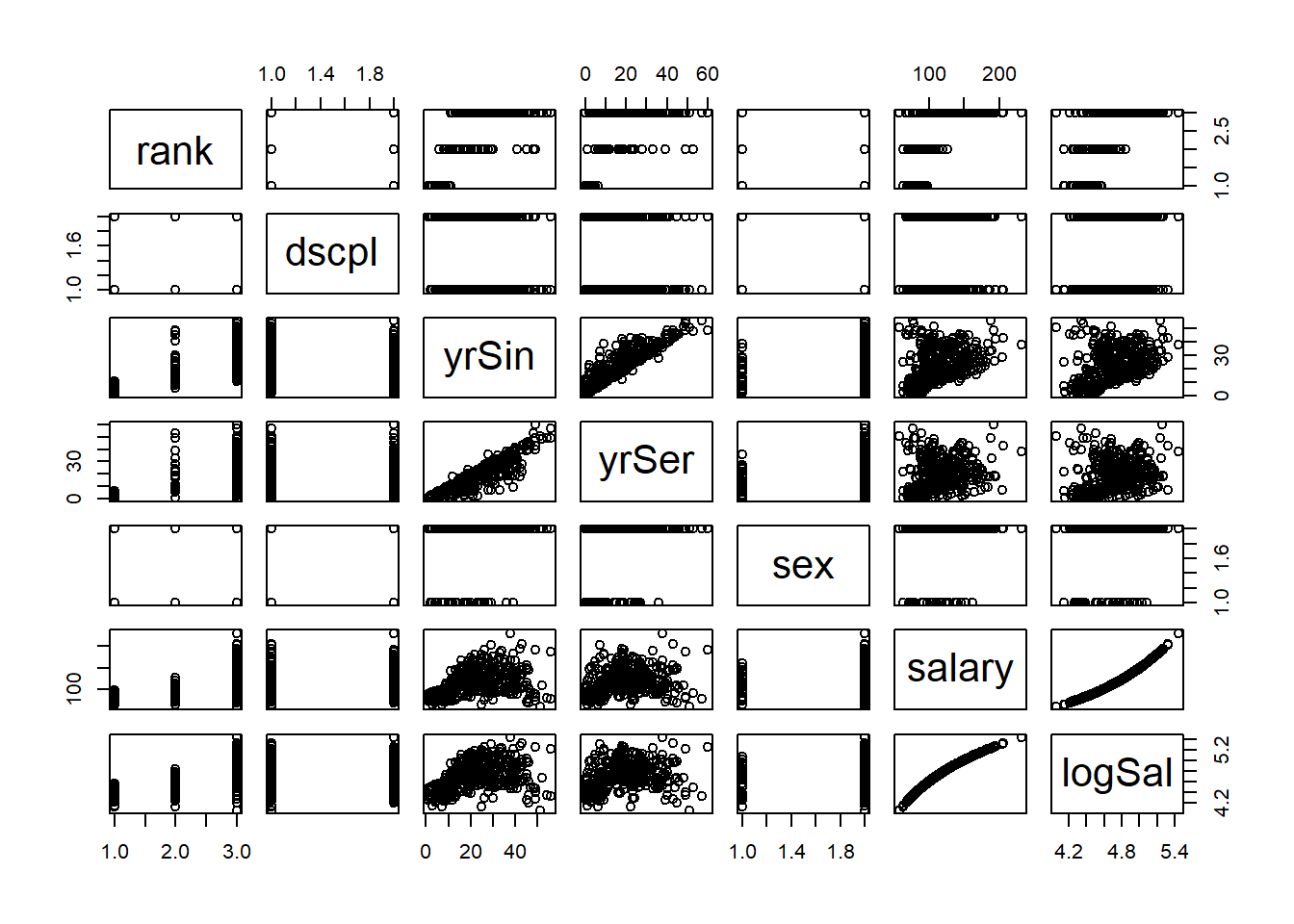Commit your changes to SalAnalysis and SalDoc files.

#### Exercise

These exercises use the alfalfa dataset and the work you started on the alfAnalysis script.

1. Use plot to create pairwise plots for the alfalfa data.frame.

2. Use ggplot to plot to create a scatter plot of the yield vs. inoc. Use a white background and color the observations based on shade level.

3. Commit your changes to AlfAnalysis.

Solutions

Next: Data presentation

Previous: Data preparation

Last Revised: 10/18/2016